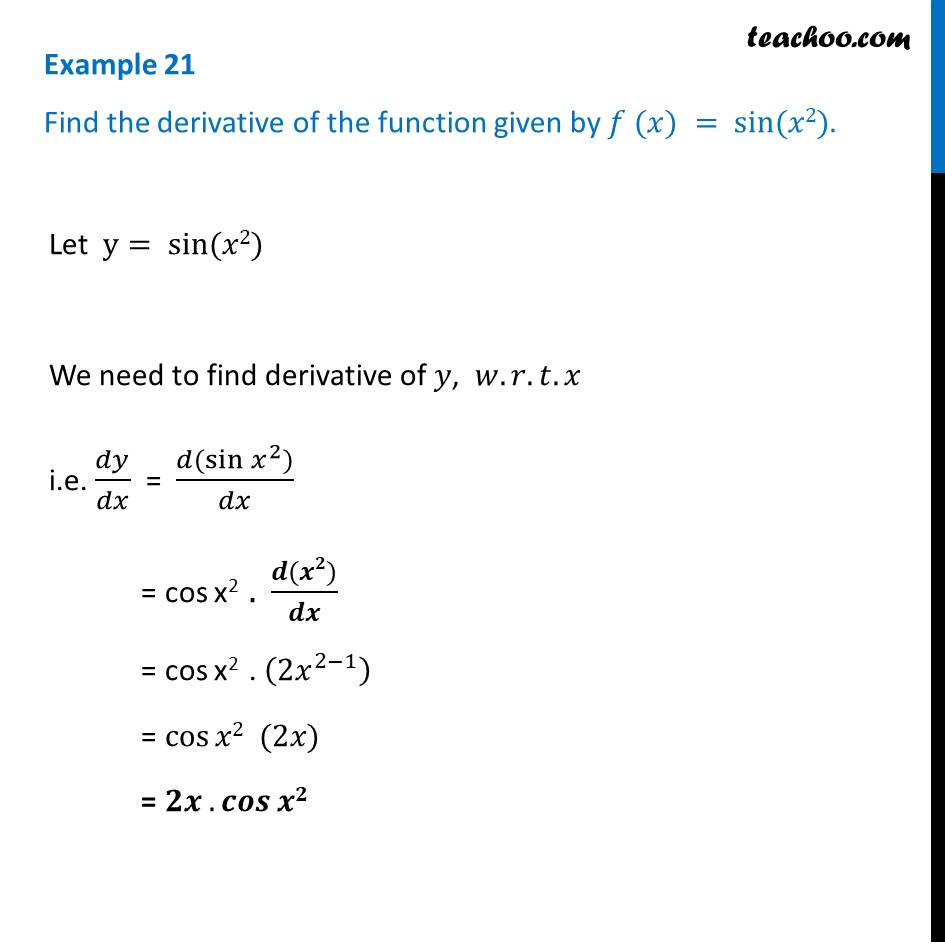Finding derivative of a function by chain rule

Chapter 5 Class 12 Continuity and Differentiability (Term 1)
Concept wise### Transcript

Example 21 Find the derivative of the function given by 𝑓 (𝑥) = sin⁡(𝑥2).Let y= sin⁡(𝑥2) We need to find derivative of 𝑦, 𝑤.𝑟.𝑡.𝑥 i.e. 𝑑𝑦/𝑑𝑥 = (𝑑(sin⁡〖𝑥^2)〗)/𝑑𝑥 = cos x2 . (𝒅(𝒙𝟐))/𝒅𝒙 = cos x2 . (〖2𝑥〗^(2−1) ) = cos⁡𝑥2 (2𝑥) = 𝟐𝒙 . 𝒄𝒐𝒔⁡𝒙𝟐